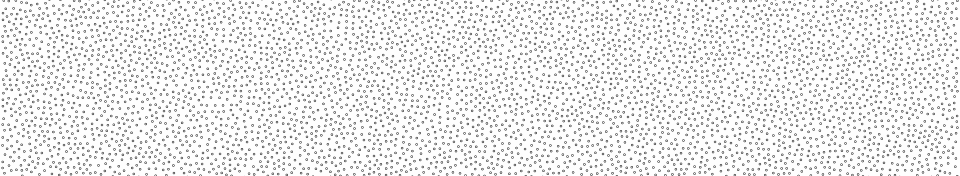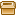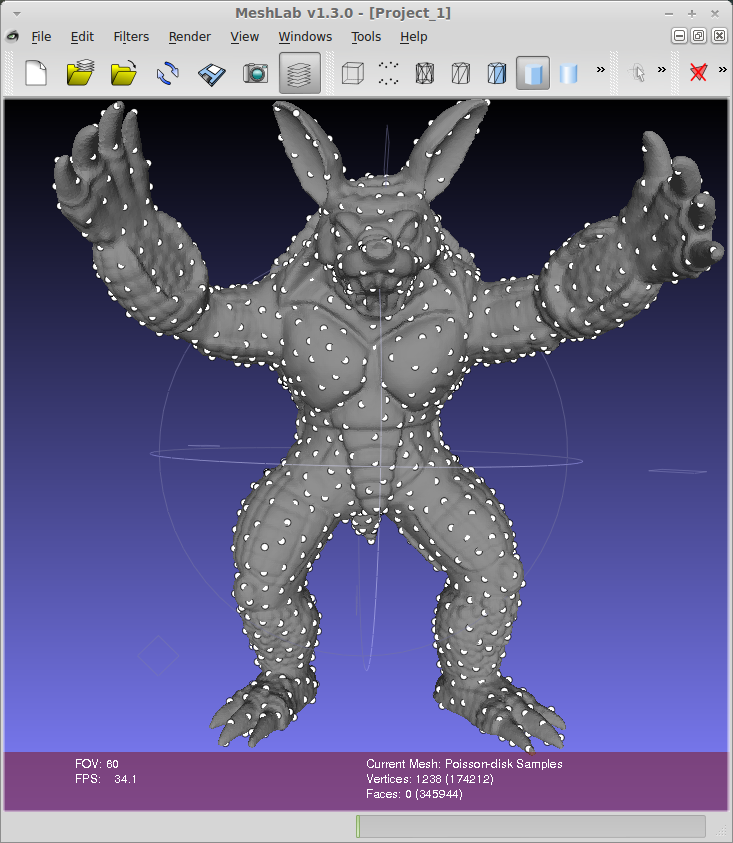# Source code for poisson disk sampling of a triangle meshHere you candownload the C++ code] to do a Poisson disk sampling of a triangular mesh. The code is a simple wrapper for the vcglib library. It takes in input a std::vector of triangles and vertex position/normals and outputs std::vector of samples positions/normals computed with the Poisson disk sampling.

EDIT: Victor Martins pointed out that the above code doesn't compile on MAC and kindly provided an [update] bundled with a newer version of vcglib.

N.B: if you are looking for a way to do Poisson disk sampling fast/in real-time take a look at this siggraph paper and matlab code. There is also a C++ implementation here.

Vcglib provides a lot of features for geometry processing and is a header library (so it's easy to install). The main drawback of the library lies in: the documentation which is not exhaustive and the heavy use of templates which makes it hard to decipher. Hopefully this wrapper will accelerate your work to implement Poisson disk sampling with vcglib.

In "poisson_disk_wrapper/utils_sampling.hpp" you'll find:

```/// @param radius : minimal radius between every samples. If radius  0 otherwise will try to match
/// and find  nb_samples by finding an appropriate radius.
/// @param verts : list of vertices
/// @param nors : list of normlas coresponding to each verts[].
/// @param tris : triangle indices in verts[] array.
/// @code
///     tri(v0;v1;v3) = { verts[ tris[ith_tri*3 + 0] ],
///                       verts[ tris[ith_tri*3 + 1] ],
///                       verts[ tris[ith_tri*3 + 2] ]   }
/// @endcode
/// @param [out] samples_pos : resulting samples positions
/// @param [out] samples_nors : resulting samples normals associated to samples_pos[]
int nb_samples,
const std::vector<Vec3>& verts,
const std::vector<Vec3>& nors,
const std::vector<int>& tris,
std::vector<Vec3>& samples_pos,
std::vector<Vec3>& samples_nor);
```

You can see the results of Poisson disk sampling with MeshLab. MeshLab implements the features of vcglib and is great to test them or see how to use them within its source code.To do the sampling:

• File ➞ Import Mesh
• Filters ➞ Sampling ➞ Poisson-Disk Sampling
• Render ➞ Show Vertex Dots

In "View ➞ Show Layer dialog" you will see the mesh and its associated samples.